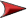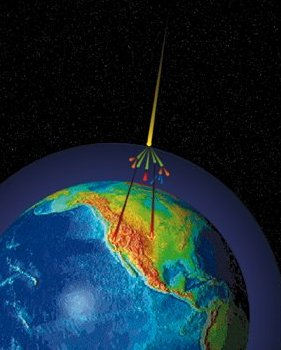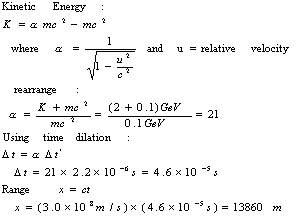# MuonsNext## Muons

Muons are the most numerous energetic charged particles at sea level. A charged particle cannot avoid losing energy by ionization. As it passes through matter the charged particle interacts with the electric fields and typically knocks loose some of the loosely bound outer electrons. A muon interacts very little with matter except by ionization. Because of this, muons can travel large distances and commonly reach the ground. However, they lose energy proportional to the amount of matter they pass. This is proportional to the density (g/cm3) times the path length (cm). This "interaction length" has units of grams per square centimeter. (see “Interaction Depth” later)

Muons lose energy at a fairly constant rate of about 2 MeV per g/cm2. Since the vertical depth of the atmosphere is about 1000 g/cm2, muons will lose about 2 GeV to ionization before reaching the ground. The mean energy of muons at sea level is still 4 GeV. Therefore the mean energy at creation is probably about 6 GeV.

The atmosphere is so tenuous at higher altitudes that even at 15,000 m it is still only 175 g/cm2 deep. Typically, it is about here that most muons are generated. Muons arrive at sea level with an average flux of about 1 muon per square centimeter per minute. This is about half of the typical total natural radiation background.

Muons (and other particles) are generated within a cone-shaped shower, with all particles staying within about 1 degree of the primary particle's path.

## Non-Vertical Muons

Muons arriving at some angle q from the vertical will have traveled a pathlength that increases as
1/cos (q). (See "Slant Depth" later.) This assumes that the Earth is essentially flat (less than 1% error for q < 70°) and that muons do not decay over the extended path length.

If we assume that twice the path length would attenuate the muons to half as many, then we would expect the muon flux to vary as the cos (q). However, the observed distribution is proportional to cos2 (q). This is a difference of less than 10% at an angle of 27° and 20% at 43°. This disparity may be primarily due to the impending decays of muons, as the path length exceeds their range.

## Relativistic Effects on MuonsThe muons have a half-life of 2.2 microseconds. At the speed of light this would give a range of only 660 m. However, at relativistic speeds, the lifetime of the muon, as we perceive it, is much longer. Given a minimal 2 GeV muon (rest mass = 0.1 GeV):

## References:

E.J. Weinberg, et.al., Physical Review D54, Particles and Fields, 1996

Particle Physics Booklet, 1994

R. Clay and B. Dawson, Cosmic Bullets, High Energy Particles in Astrophysics, A-W 1997

M. Shapiro, Cosmic Radiation in Contemporary Astrophysics, Reidel, 1986

D. Montgomery, Cosmic Ray Physics, Princeton, 1949

F. Delobeau, The Environment of the Earth, Reidel, 1971

National Geophysical Data Center, NOAA, Cosmic Rays, http://web.ngdc.noaa.gov/stp/SOLAR/COSMIC_RAYS/cosmic.html

National Geophysical Data Center, NOAA, U.S. Standard Atmosphere 1976, http://nssdc.gsfc.nasa.gov/space/model/atmos/us_standard.html

I. Kroo, Aircraft Aerodynamics, Stanford, Standard Atmosphere Calculations, http://aero.stanford.edu/StdAtm.html